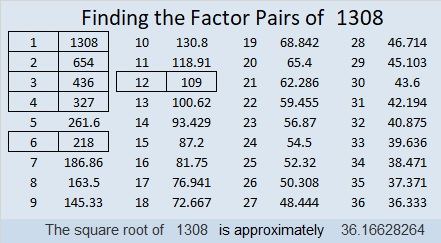# 1308 and Level 6

Can you find the factors that will turn this puzzle into a multiplication table? It’s a level 6 so you might find it to be a challenge. Use logic. Don’t guess and check.Print the puzzles or type the solution in this excel file: 10-factors-1302-1310

Here are a few facts about the puzzle number, 1308:

• 1308 is a composite number.
• Prime factorization: 1308 = 2 × 2 × 3 × 109, which can be written 1308 = 2² × 3 × 109
• The exponents in the prime factorization are 2, 1, and 1. Adding one to each and multiplying we get (2 + 1)(1 + 1)(1 + 1) = 3 × 2 × 2 = 12. Therefore 1308 has exactly 12 factors.
• Factors of 1308: 1, 2, 3, 4, 6, 12, 109, 218, 327, 436, 654, 1308
• Factor pairs: 1308 = 1 × 1308, 2 × 654, 3 × 436, 4 × 327, 6 × 218, or 12 × 109
• Taking the factor pair with the largest square number factor, we get √1308 = (√4)(√327) = 2√327 ≈ 36.166281308 is the hypotenuse of a Pythagorean triple:
720-1092-1308 which is 12 times (60-91-109)

As shown in their factor trees below, 1308, 1309, 1310, and 1311 each have three distinct prime numbers in their prime factorizations. They are the smallest set of four consecutive numbers with the same number of prime factors. Thank you OEIS.org for alerting me to that fact.This site uses Akismet to reduce spam. Learn how your comment data is processed.